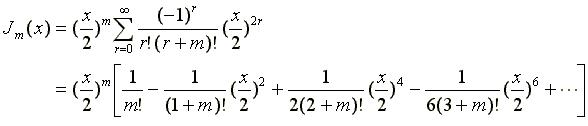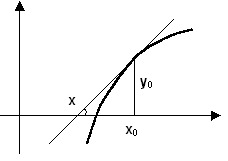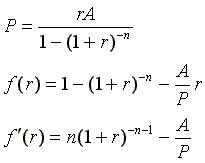# LAB 7

## Objectives

• Explore Numerical Methods
• Handle non-closed-form functions
• Realize the limitation of the single-program approach

## Task-A: Charting an Infinite Series

Some functions have no closed-form formula that computes `y` at a given `x` in terms of standard functions and operators. One example is the Bessel function of the first kind, defined as:For every non-negative integer value of `m`, we get a different Bessel function so the above series defines in fact a family of Bessel functions. These functions appear as solutions to integral and differential equations in diverse scientific fields and are considered as natural extensions of trigonometric functions like `sin` and `cos`. In this Lab, we focus on `J1(x)`, the Bessel function of the first kind of order 1 (obtained by substituting `m = 1` in the above) and seek to chart it in the range [0,10].

1. Rewrite the above sum for `m = 1` and write out the first 6 or seven terms in the series. Try to see a pattern that allows one to compute each term from its predecessor; e.g. ask yourself: if I was told that the 4th term is 0.725 and was given the value of x, how do I compute the 5th term? You can, of course, compute the 5th term from scratch but we prefer an incremental approach.
2. Write a program that prompts for a read the value of `x`, a `real*8` number and compute the sum of the series. The loop should keep computing and accumulating terms until the absolute value of the term to be added becomes less than some `EPSILON`. Make sure that all computations are done using `real*8` and/or `integer*2`; i.e. do not allow `real*4` round-off errors.
3. Set `EPSILON` to `1.E-3`.
4. Run the program and compute `J1(0)`. The answer should be `0`, and if not, debug your program.
5. Run the program and compute `J1(10)`. The answer should be about `0.04`; if not, debug your program.
6. Modify the program so it also outputs the term count; i.e. the number of terms that were added before the summation stopped. Re-run the previous two cases and justify the displayed term counts.
7. How do your answers change if `EPSILON` is changed to `1.E-6`?
8. How do your answers change if `EPSILON` is changed to `1.E-15`?
9. Modify the program so it does not prompt for `x`. Instead, it sets up a loop that generates 21 values for `x`, ranging from `0` to `10` in increments of `0.5`. The output must show the value of `x` and the corresponding value of `J1(x)` in a tabular fashion; e.g.
```       0.0   0.000000000
0.5   0.242268458
...
...
9.5   0.161264431
10.0   0.043472746```
(use a small value of `EPSILON`, like 1.E-12, and format the output so that 9 decimals are shown.)
10. Modify the program so that the tabulation is not done on the screen but on a disk file named `bessel.txt`.
11. Launch Microsoft Excel and in it, open the file `bessel.txt`. Note that Excel can read multi-column text files if they have fixed sizes (the columns are aligned) or if they are delimited (a space or a tab separates the columns). Our file has fixed-size columns that are space-delimited, and hence, Excel can read it either way it sees it.
12. Once opened, highlight all the shown values and choose `Chart` from the `Insert` menu. Specify that you want an XY (scatter) chart, and choose one which connects the points by smooth lines.
13. The remaining options can be set based on your own preference but place the chart in a sheet by itself (rather than mixed with the data).
14. Deliverables: printed program, printout of the tabulation, a hard copy of the chart, and the answers to the above explorations.

## Task-B: Zeros of a non-linear function

Given a function `f(x)`, and a value `x0` near which the function is believed to vanish, Newton's method allows us to quickly determine the value `x*` at which `f(x*) = 0`. The method works by following the tangent of the curve of `f(x)` and computing its x-intercept. Starting with `x0`, this leads to successive approximations of the form:```x = x0 - f(x0) / f'(x0) ```

which converges to the sought zero (if one exists). Implementing this algorithm involves a loop that computes successive approximations until the abs value of the function at a computed `x` becomes less than `EPSILON` or the difference (in abs value) between two successive approximations is less than `EPSILON`. To guard against situations in which there is no zero, or the zero is not reachable from the starting point, we also stop the loop (and declare failure in finding the zero) if more than 1000 iterations were performed.

We will apply the above technique for solving the following problem: You need to buy a house whose present value is A=\$135,000. You don't have that much in cash but you can apply for a 30-year mortgage and make monthly payments. The maximum you can pay each month is \$1000. What should the annual interest rate be so that you can afford this house?

We have already seen the mortgage formula, which relates the cash price `A`, the amortization period `n`, the monthly interest percentage `r`, and the monthly payment `P`, but the unknown now is `r`. We are therefore interested in finding the zero of the function `f(r)`, given by:1. Write a program that implements Newton's method for a function whose zero is known (e.g. `(x2-1)`). When run, the program prompts for an initial value `x0` and then either outputs the sought zero or state that no zero was found.
2. Set `EPSILON` to `1.E-3`. and run. Did you get the correct zero of the function? If not, debug your program.
3. How does your answer change if `EPSILON` is changed to `1.E-6`?
4. Modify the program so it also outputs an iteration count; i.e. the number of repeated applications of Newton's formula before the iteration stopped. Re-run the previous two cases (different `EPSILON` values) and justify the shown counts.
5. Does the iteration count depend also on the initial guess `x0`?
6. Modify the function to one that is known not to have zeros; e.g. `(x2+1)`. Did your program get stuck in an infinite loop or did it correctly detect the problem?
7. Modify the program so it locates the zero of the function `f(r)` defined above. Note that the independent variable is now called `r`, not `x`, so you need to rename one to the other.
8. Modify the program so it displays the zero as an annual percent (rather than a monthly percentage) formatted to one decimal.
9. Deliverables: printed program and the answers to the above explorations.

Fall2002/HR